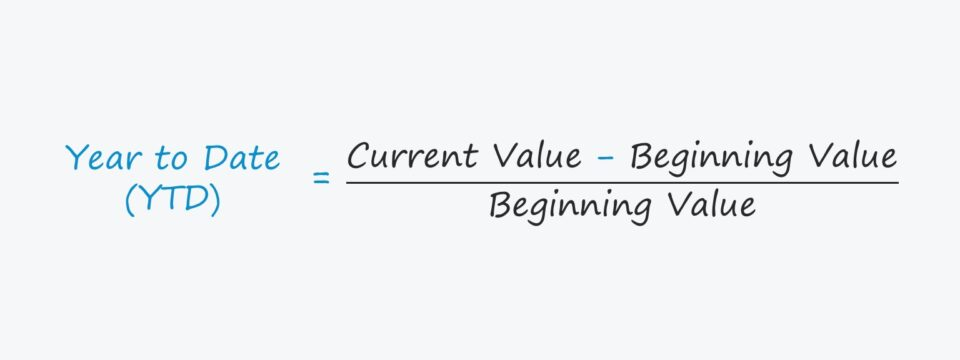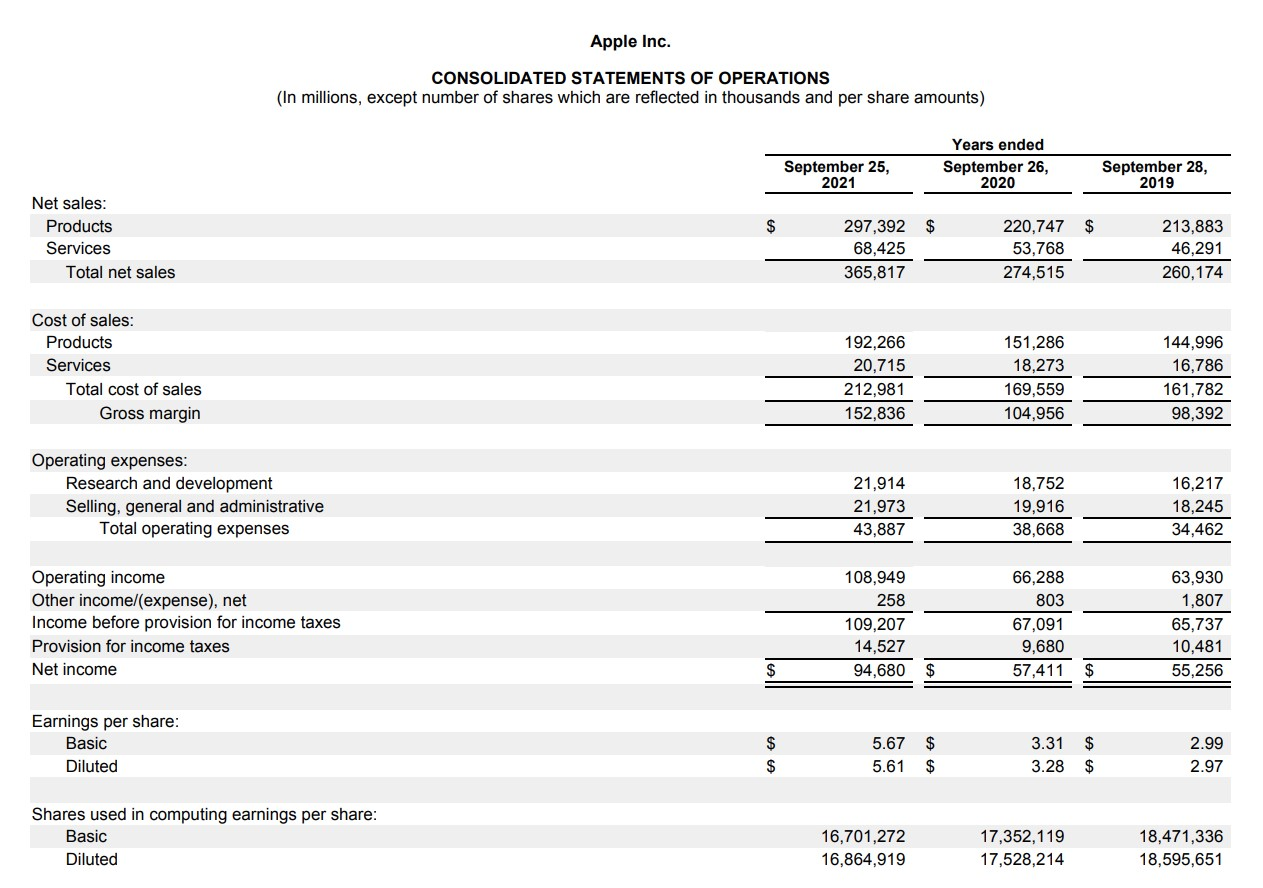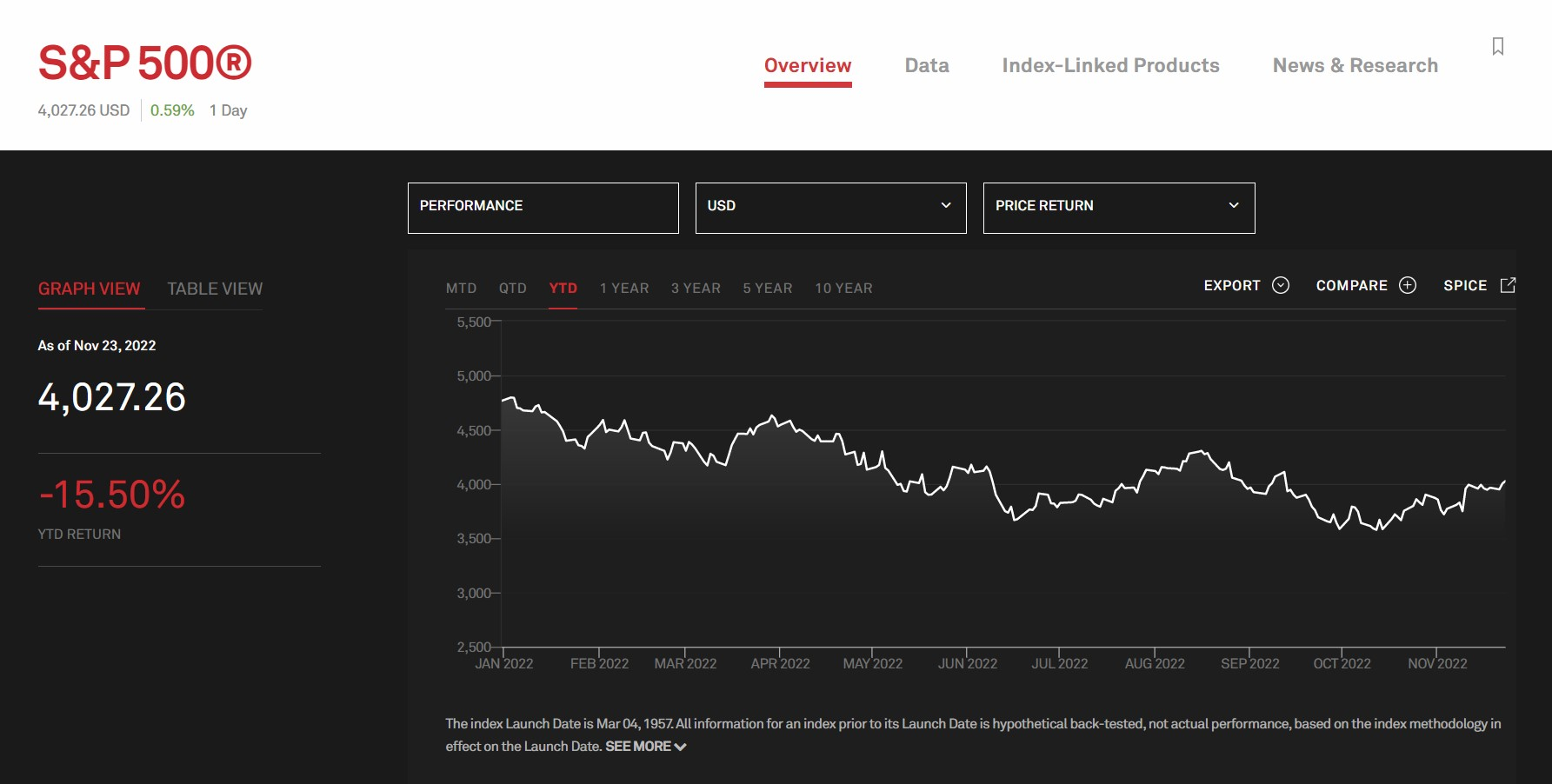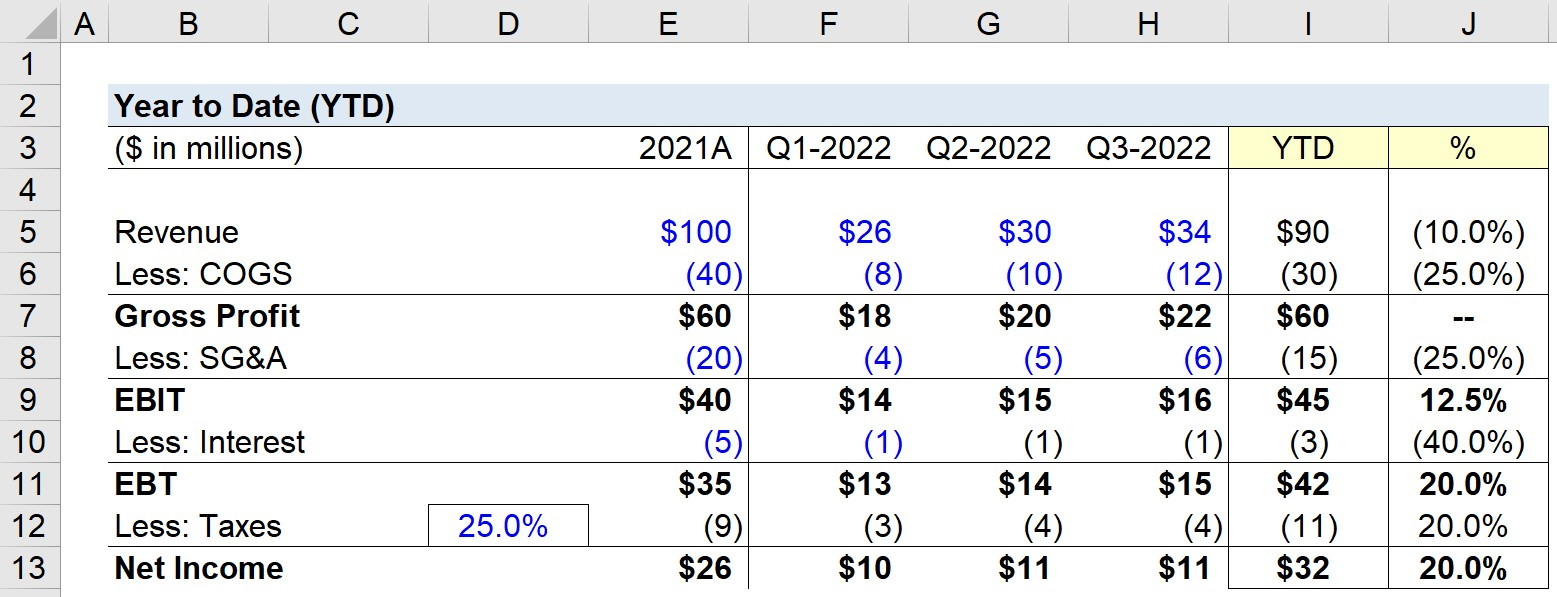Welcome to Wall Street Prep! Use code at checkout for 15% off.# Year to Date (YTD)

Guide to Understanding Year to Date (YTD)## What Does YTD Mean?

In finance, the term YTD stands for “Year to Date” and refers to the period starting from the first date of the current year to the current period.

However, the YTD abbreviation could also mean the period between the beginning date of the fiscal year to the current date, or the most recent reporting period, such as the latest quarterly report.

In that case, the year-to-date (YTD) is most likely alluding to the financial statements of the company, rather than a percentage return.

By measuring YTD performance, a company can assess its performance to date and determine how its current trajectory compares to its prior periods and internal forecasts, as well as for benchmarking purposes with comparable companies in either the same or an adjacent industry.

The trend of the company’s sales performance, or alternatively the returns on a portfolio, can be useful for understanding the current state of its performance and if adjustments are necessary in order to reach the goals set by the management team.

## How to Calculate YTD?

Suppose the management team of Microsoft (MSFT) were to state that their share price is currently up over 40% YTD.

Therefore, as of the current date in August 2023, MSFT’s stock price has increased over 40% since January 1, 2023.

To calculate the YTD return, subtract the starting period value from the current period value, and divide the resulting figure by the starting year value.

In the final step, multiply the figure in decimal notation by 100 to convert the YTD figure into a percentage.

For most companies, the starting date of the fiscal year tends to be January 1st, however, there are exceptions – such as companies such as Apple (AAPL) with fiscal years that begin on different dates, i.e. the end of September.Apple Fiscal Year Ending Date Example (Source: Apple 10-K)

## YTD Formula

The formula to calculate year-to-date (YTD) performance or returns is as follows.

Year to Date (YTD) = [(Current Period Value Beginning of Period Value)] ÷ Beginning of Period Value)

## YTD Returns Calculation Example

In order to convert the decimal value into a percentage, the resulting figure must be multiplied by 100.

For example, if an investor’s portfolio was worth \$200,000 at the beginning of 2022 and is currently worth \$220,000 in the middle of 2022, the year-to-date return is calculated as 10%.

• Year to Date (YTD) = [(\$220,000 – \$200,000) ÷ \$200,000) = 0.10, or 10%

## S&P 500 YTD Returns Graph

The S&P 500, or “Standard and Poor’s 500”, is a stock market index tracking the performance of approximately 500 publicly-traded companies based in the U.S.

The screenshot of the graph below reflects the YTD returns of the S&P 500 index as of the latest closing date, November 23, 2022.S&P 500 Index YTD Returns (Source: S&P Dow Jones Indices)

## YTD Calculator

We’ll now move to a modeling exercise, which you can access by filling out the form below.Submitting...

## 1. Operating Assumptions

Suppose a company is measuring its year-to-date financial performance to compare its revenue and earnings figures to that of its last fiscal year, 2021.

The company’s 2021 fiscal year and quarterly income statement metrics are as follows.

Income Statement 2021A Q1-2022 Q2-2022 Q3-2022
Revenue \$100 million \$26 million \$30 million \$34 million
Less: COGS (40) million (8) million (10) million (12) million
Gross Profit \$60 million \$18 million \$20 million \$22 million
Less: SG&A (20) million (4) million (5) million (6) million
EBIT \$40 million \$14 million \$15 million \$16 million
Less: Interest (5) million (1) million (1) million (1) million
EBT \$35 million \$13 million \$14 million \$15 million
Taxes (@ 25% Tax Rate) (9) million (3) million (4) million (4) million
Net Income \$26 million \$10 million \$11 million \$11 million

## Step 2. YTD Financials Calculation Example

By taking the sum of the quarterly figures, we can arrive at our company’s 2022 year-to-date metrics.

Q1 to Q3 2022 Financials

• Revenue = \$90 million
• COGS = (30) million
• Gross Profit = \$60 million
• SG&A = (15) million
• EBIT = \$45 million
• Interest = (3) million
• EBT = \$42 million
• Taxes = (11) million
• Net Income = \$32 million

Note that year-to-date (YTD) financials could also refer to the last four quarters. If that is the case, we would add the financials from Q4-2021.

But in our modeling exercise, we’re attempting to gauge the progress of only Q-1 to Q-3 2022 performance to determine how it is tracking compared to fiscal year 2021.

By comparing the performance of three quarters to a full fiscal year, a company can quantify the difference to set targets for Q-4 2022 performance.

## 3. YTD Revenue and Earnings Calculation Example

If we divide the ending values from above by the beginning values (2021A), we can determine how the company is performing to date.

We’ll focus on the company’s revenue and earnings metrics:

• Revenue (%) → We can see that the company’s revenue is currently only off by 10% (with one more quarter to go) and thus should easily surpass its 2021 amount (\$90 million vs. \$100 million).
• Gross Profit (%) → Next, the amount of gross profits generated in the first three quarters of 2022 is equivalent to the total gross profit in 2021 (\$60 million vs. \$60 million).
• EBIT (%) → The operating income, or “EBIT”, from the first three quarters has already exceeded the 2021 amount by approximately 12.5% (\$45 million vs. \$50 million).
• Net Income (%) → Finally, the company’s year to date net income, i.e. the “bottom line”, is up by roughly 20% (\$32 million vs. \$26 million).Step-by-Step Online Course

### Everything You Need To Master Financial Modeling

Enroll in The Premium Package: Learn Financial Statement Modeling, DCF, M&A, LBO and Comps. The same training program used at top investment banks.

Inline Feedbacks
samaneh
May 5, 2023 10:32 am

hi,i want spot formol:
y~=(cp/p0)+(par-p0/T)

May 7, 2023 9:03 pm

Hi, Samaneh,

BBLearn Financial Modeling Online

Everything you need to master financial and valuation modeling: 3-Statement Modeling, DCF, Comps, M&A and LBO.

The Wall Street Prep Quicklesson Series

### 7 Free Financial Modeling Lessons

Get instant access to video lessons taught by experienced investment bankers. Learn financial statement modeling, DCF, M&A, LBO, Comps and Excel shortcuts.